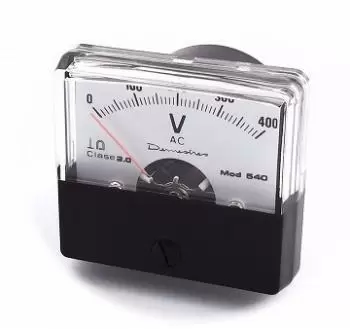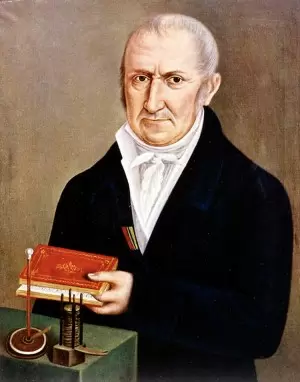# What Is a Volt? Tension UnitThe volt is the unit of the international system measured to measure the voltage and electromotive force.

The volt is represented by the symbol V. The measuring instrument for measuring voltage is the voltmeter.

The electrical potential difference can be imagined as the ability to move electrical charges through a resistor. Essentially, the volt measures the amount of kinetic energy that each electron carries. The number of electrons is measured as electric charge, in coulombs.

The volt can be multiplied by the current flow, measured in amps. The result is the total electric potential of the current. It is measured in watts. Amps are coulombs per second.

The difference in electric potential was called the electromotive force at a time when the word force was used in the world of physics with little care. Today this term is still used in some context.

The etymology of the name volt is derived from Alessandro Volta. Volta invented the voltaic cell in 1799, the first electrochemical battery.

In the 1880s, the International Congress on Electricity, now the International Electrotechnical Commission (IEC), approved the volt as a unit of measurement for electromotive force.

## Volt Definition

Two different definitions of volt can be given:

On the one hand, we can consider that a volt is the voltage existing between two points of a conductor through which a current of one ampere (A) passes and a power of one watt (W) is dissipated.

Another alternative way of defining it: The volt is equivalent to the potential difference that exists between two points of a conductor when when transporting a coulomb between them (C), the work of a july is performed (J).

### Definition Through the Josephson Effect

At one point the definition of volts was different. A volt was calculated through the very precise relationship between frequency and volt with a cesium clock as the time reference.

This happened with the help of a Josephson crossing. The Josephson junction consists of two superconductors that are connected by an insulator.

This relationship turned out to be independent of the arrangement or materials used. Therefore, it is extremely accurate.

However, it is no longer the official method according to the International System of Units.

## Example Comparing It with the Flow of Water

One way to understand how an electric current works is by using the analogy of water flow.

If the circuits were water pipes we would have to:

• Voltage (difference in electrical potential) would be difference in water pressure.

• The electric current would be proportional to the diameter of the pipe or the amount of water flowing at that pressure.

• A resistance would be a small diameter somewhere in the pipe.

• A capacitor / inductor could be compared to a "U" shaped pipe. In this "U" a higher water level on one side could temporarily store energy.

## Voltage and Ohm's Law

The relationship between voltage and current is defined by Ohm's law. Ohm's law is analogous to the Hagen-Poiseuille equation, since both are linear models that relate the flow and the potential in their respective systems.

Ohm's law states that the potential difference V between two points of a conductor is proportional to the intensity of the current flowing through it.

## Volt History

This unit is named in honor of Alessandro Volta, who in 1800 invented the voltaic cell, the first chemical battery.Alessandro Volta, was an Italian physicist. In 1800 Volta announced the results of his experiments since 1786 at the Royal Society of London.

With the experiments, Alessandro Volta generated electricity using different metals separated by a wet conductor. Alsessandro Volta stacked 30 metal discs each separated by a cloth moistened with salt water, thus, the Italian physicist obtained electricity in the form of direct current.

Such a device was called a "voltaic battery". The name of the batteries and the unit of measurement of electric potential came from the voltaic cell: the volt.

## Summary

A volt is the unit of the international system of measurements to express a difference in electrical potential.

The value of a volt indicates the current intensity of one ampere (A) between two points and that a power of one watt (W) is dissipated.

Author:

Published: May 13, 2015
Last review: May 29, 2020